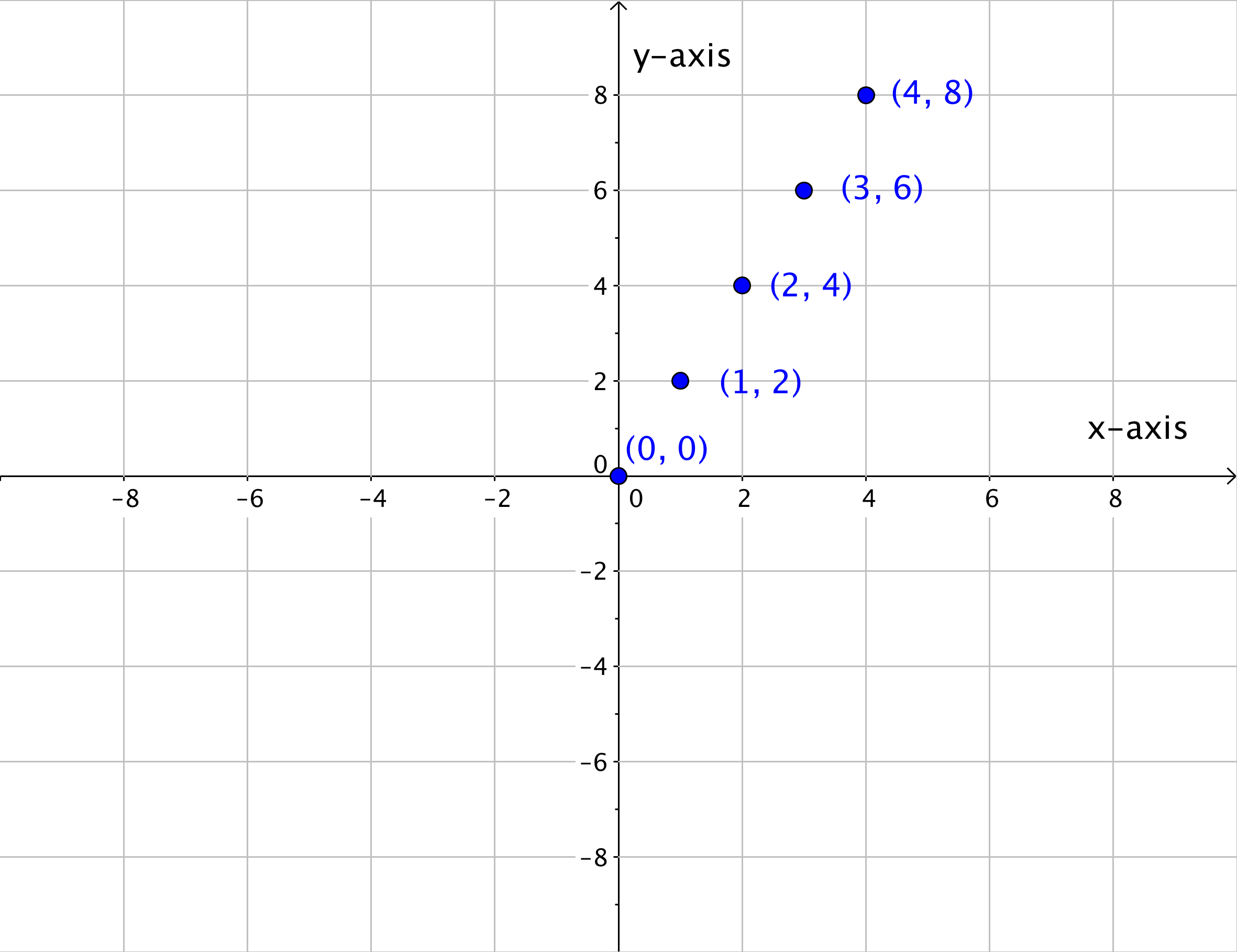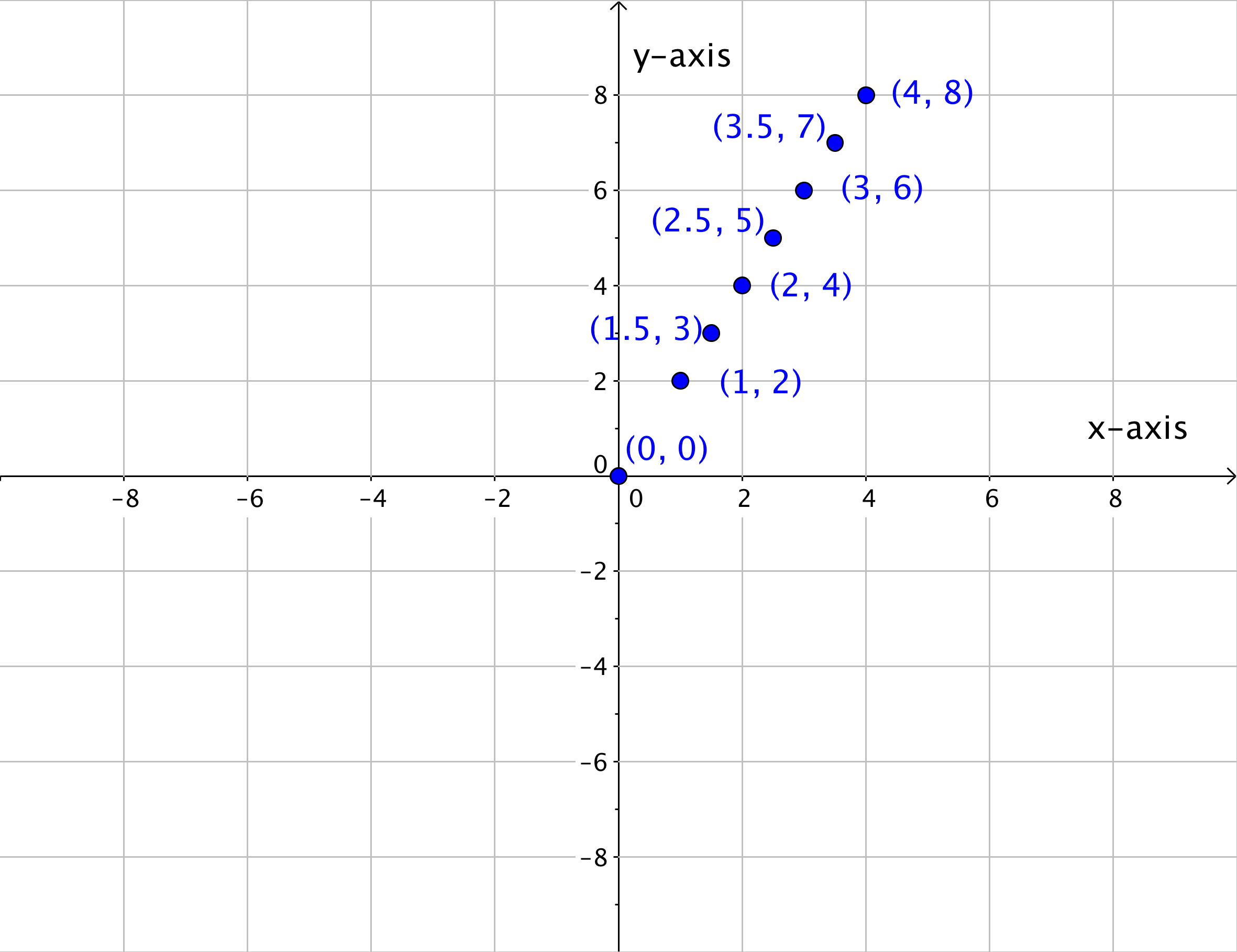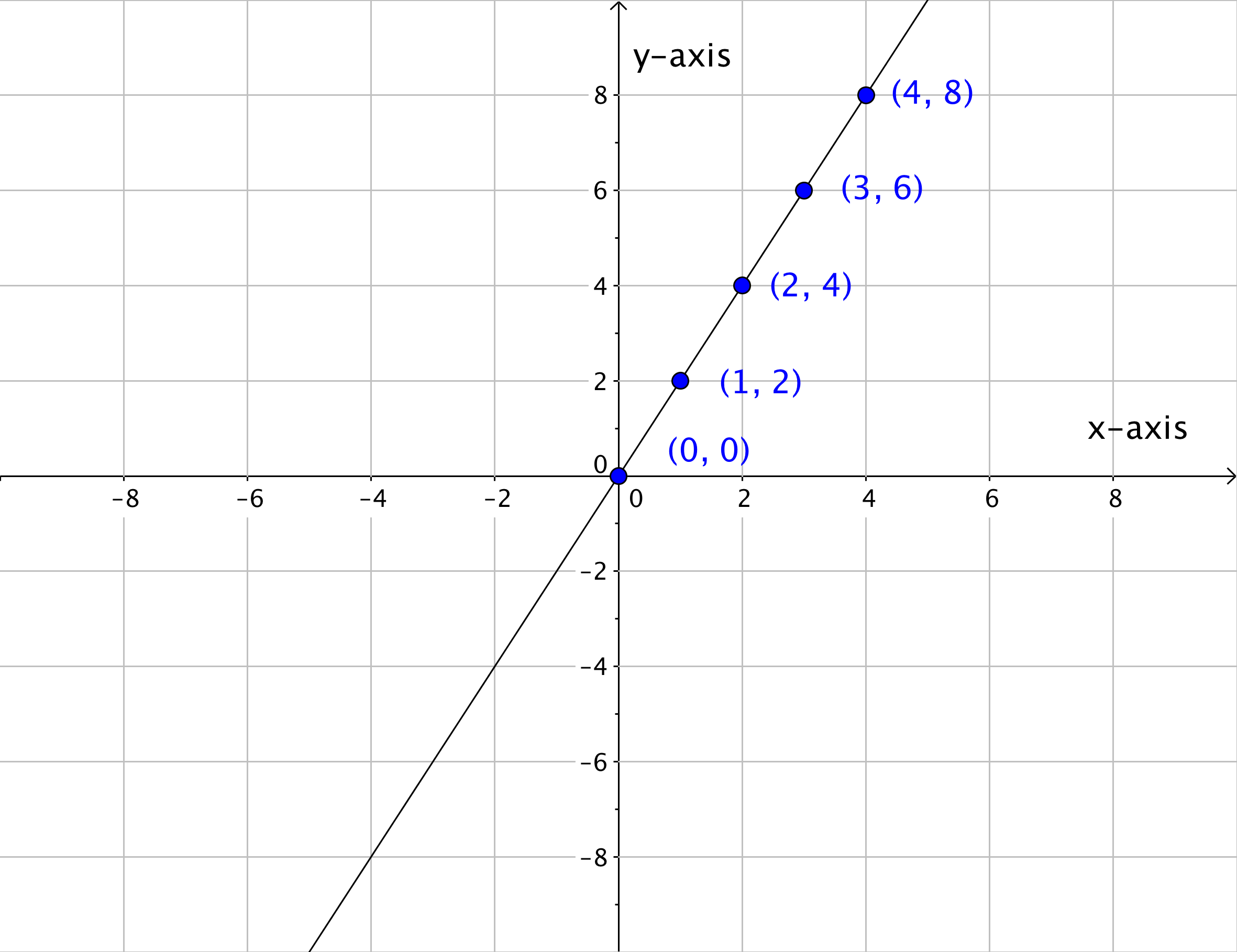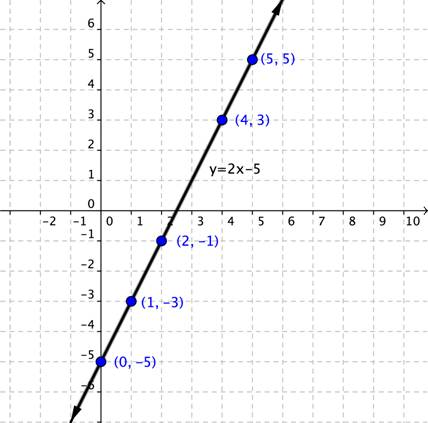## Graphing Linear Equations Using Ordered Pairs

### Learning Outcomes

• Determine whether an ordered pair is a solution of an equation
• Complete a table of solutions for a linear equation
• Graph linear equations in different forms using ordered pairs

Graphing ordered pairs is only the beginning of the story. Once you know how to place points on a grid, you can use them to make sense of all kinds of mathematical relationships.

You can use a coordinate plane to plot points and to map various relationships, such as the relationship between an object’s distance and the elapsed time. Many mathematical relationships are linear relationships. Let’s look at what a linear relationship is.

## Plotting points to graph linear relationships

A linear relationship is a relationship between variables such that when plotted on a coordinate plane, the points lie on a line. Let’s start by looking at a series of points in Quadrant I on the coordinate plane.

Look at the five ordered pairs (and their x– and y-coordinates) below. Do you see any pattern to the location of the points? If this pattern continued, what other points could be on the line?

You probably identified that if this pattern continued the next ordered pair would be at $(5, 10)$. This makes sense because the point $(5, 10)$ “lines up” with the other points in the series—it is literally on the same line as the others. Applying the same logic, you may identify that the ordered pairs  $(6, 12)$ and $(7, 14)$ would also belong if this coordinate plane were larger; they, too, will line up with the other points.

These series of points can also be represented in a table. In the table below, the x- and y-coordinates of each ordered pair on the graph is recorded.

 x-coordinate y-coordinate $0$ $0$ $1$ $2$ $2$ $4$ $3$ $6$ $4$ $8$

Notice that each y-coordinate is twice the corresponding x-value. All of these x- and y-values follow the same pattern, and, when placed on a coordinate plane, they all line up.Once you know the pattern that relates the x- and y-values, you can find a y-value for any x-value that lies on the line. So if the rule of this pattern is that each y-coordinate is twice the corresponding x-value, then the ordered pairs $(1.5, 3), (2.5, 5)$, and $(3.5, 7)$ should all appear on the line too, correct? Look to see what happens.If you were to keep adding ordered pairs (x, y) where the y-value was twice the x-value, you would end up with a graph like this.Look at how all of the points blend together to create a line. You can think of a line, then, as a collection of an infinite number of individual points that share the same mathematical relationship. In this case, the relationship is that the y-value is twice the x-value.

There are multiple ways to represent a linear relationship—a table, a linear graph, and there is also a linear equation. A linear equation is an equation with two variables whose ordered pairs graph as a straight line.

There are several ways to create a graph from a linear equation. One way is to create a table of values for x and y, and then plot these ordered pairs on the coordinate plane. Two points are enough to determine a line. However, it’s always a good idea to plot more than two points to avoid possible errors.

Then you draw a line through the points to show all of the points that are on the line. The arrows at each end of the graph indicate that the line continues endlessly in both directions. Every point on this line is a solution to the linear equation.

### Example

Graph the linear equation $y=−1.5x$.

## Graph the linear equation

### Example

Graph the linear equation $y=2x+3$.

## Ordered Pairs as Solutions

So far, you have considered the following ideas about lines: a line is a visual representation of a linear equation, and the line itself is made up of an infinite number of points (or ordered pairs). The picture below shows the line of the linear equation $y=2x–5$ with some of the specific points on the line.Every point on the line is a solution to the equation $y=2x–5$. You can try any of the points that are labeled like the ordered pair, $(1,−3)$.

$\begin{array}{l}\,\,\,\,y=2x-5\\-3=2\left(1\right)-5\\-3=2-5\\-3=-3\\\text{This is true.}\end{array}$

You can also try ANY of the other points on the line. Every point on the line is a solution to the equation $y=2x–5$. All this means is that determining whether an ordered pair is a solution of an equation is pretty straightforward. If the ordered pair is on the line created by the linear equation, then it is a solution to the equation. But if the ordered pair is not on the line—no matter how close it may look—then it is not a solution to the equation.

### Identifying Solutions

To find out whether an ordered pair is a solution of a linear equation, you can do the following:

• Graph the linear equation, and graph the ordered pair. If the ordered pair appears to be on the graph of a line, then it is a possible solution of the linear equation. If the ordered pair does not lie on the graph of a line, then it is not a solution.
• Substitute the (x, y) values into the equation. If the equation yields a true statement, then the ordered pair is a solution of the linear equation. If the ordered pair does not yield a true statement then it is not a solution.

### Try It

Watch the video below to see more about how solutions to linear equations lie on their graphs.

## Solve for y, then graph a linear equation

The linear equations we have graphed so far are in the form $y=mx+b$ where m and b are real numbers. In this section we will graph linear equations that appear in different forms than we have seen.

TIP:  You can use ANY values for x that fit on your graph!  Pick easy numbers to work with, too!

### Example

Graph the linear equation $y+3x=5$.

## Horizontal and Vertical Lines

The linear equations $x=2$ and $y=−3$ only have one variable in each of them. However, because these are linear equations, then they will graph on a coordinate plane just as the linear equations above do. Just think of the equation $x=2$ as $x=0y+2$ and think of $y=−3$ as $y=0x–3$.

### Example

Graph $y=−3$.

Watch the video below for more examples of how to graph horizontal and vertical lines.

## Contribute!

Did you have an idea for improving this content? We’d love your input.